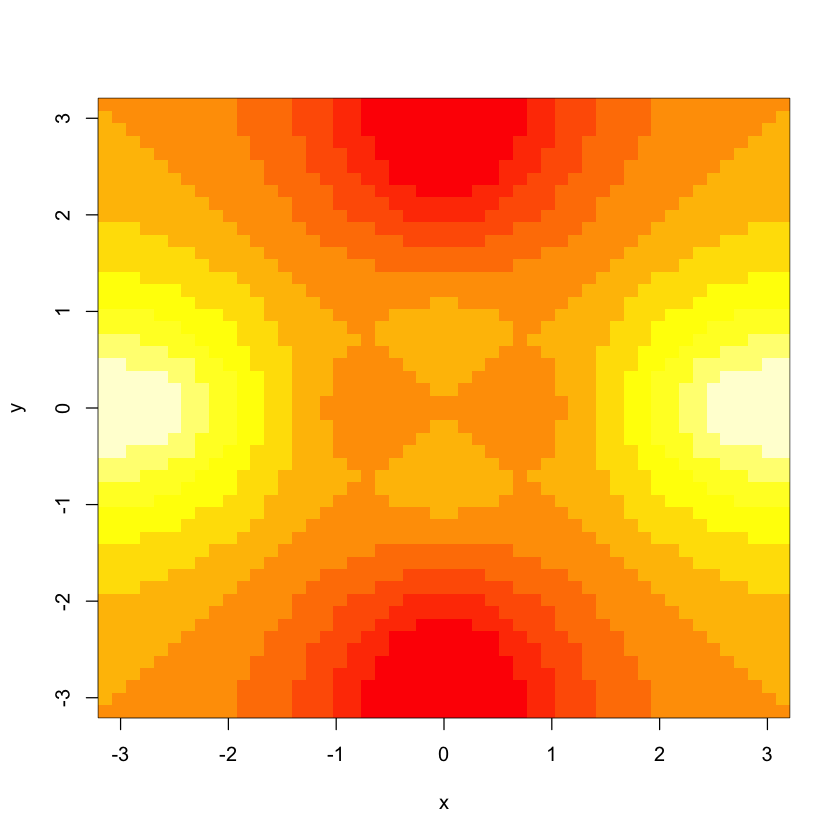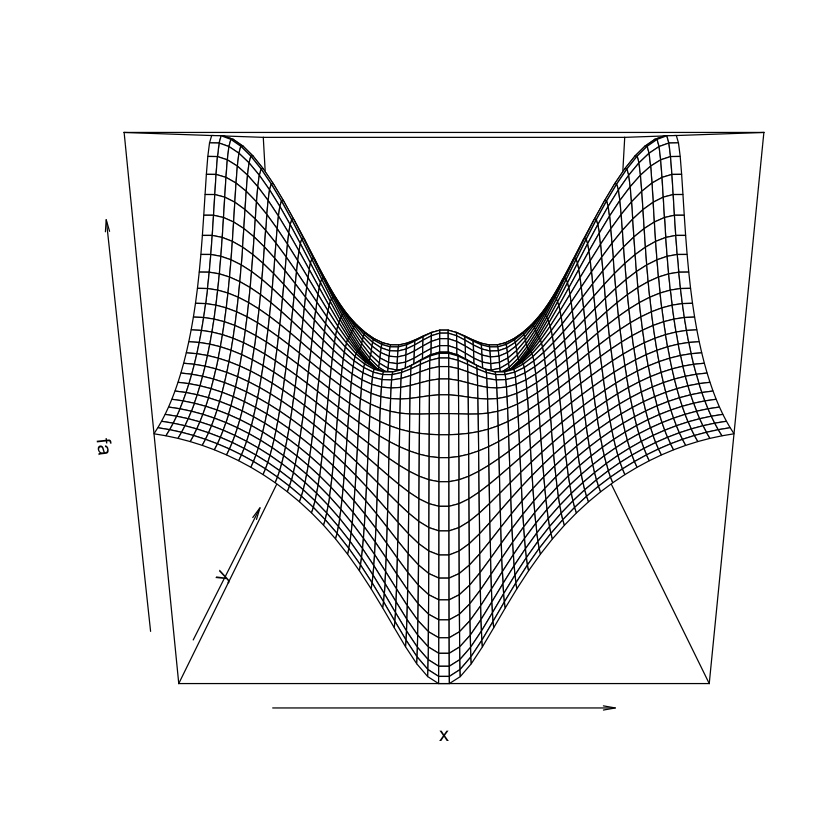In :
# Chapter 2 Lab: Introduction to R

# Basic Commands

x <- c(1,3,2,5)
x

1. 1
2. 3
3. 2
4. 5
In :
x = c(1,6,2)
x

1. 1
2. 6
3. 2
In :
y = c(1,4,3)
length(x)
length(y)

3
3
In :
x+y

1. 2
2. 10
3. 5
In :
ls()

1. 'A'
2. 'Auto'
3. 'cylinders'
4. 'df'
5. 'f'
6. 'fa'
7. 'q'
8. 'quit'
9. 'x'
10. 'y'
In :
rm(x,y)
ls()

1. 'A'
2. 'Auto'
3. 'cylinders'
4. 'df'
5. 'f'
6. 'fa'
7. 'q'
8. 'quit'
In :
rm(list=ls())

In :
?matrix

 matrix {base} R Documentation

## Matrices

### Description

matrix creates a matrix from the given set of values.

as.matrix attempts to turn its argument into a matrix.

is.matrix tests if its argument is a (strict) matrix.

### Usage

matrix(data = NA, nrow = 1, ncol = 1, byrow = FALSE,
dimnames = NULL)

as.matrix(x, ...)
## S3 method for class 'data.frame'
as.matrix(x, rownames.force = NA, ...)

is.matrix(x)


### Arguments

 data an optional data vector (including a list or expression vector). Non-atomic classed R objects are coerced by as.vector and all attributes discarded. nrow the desired number of rows. ncol the desired number of columns. byrow logical. If FALSE (the default) the matrix is filled by columns, otherwise the matrix is filled by rows. dimnames A dimnames attribute for the matrix: NULL or a list of length 2 giving the row and column names respectively. An empty list is treated as NULL, and a list of length one as row names. The list can be named, and the list names will be used as names for the dimensions. x an R object. ... additional arguments to be passed to or from methods. rownames.force logical indicating if the resulting matrix should have character (rather than NULL) rownames. The default, NA, uses NULL rownames if the data frame has ‘automatic’ row.names or for a zero-row data frame.

### Details

If one of nrow or ncol is not given, an attempt is made to infer it from the length of data and the other parameter. If neither is given, a one-column matrix is returned.

If there are too few elements in data to fill the matrix, then the elements in data are recycled. If data has length zero, NA of an appropriate type is used for atomic vectors (0 for raw vectors) and NULL for lists.

is.matrix returns TRUE if x is a vector and has a "dim" attribute of length 2) and FALSE otherwise. Note that a data.frame is not a matrix by this test. The function is generic: you can write methods to handle specific classes of objects, see InternalMethods.

as.matrix is a generic function. The method for data frames will return a character matrix if there is only atomic columns and any non-(numeric/logical/complex) column, applying as.vector to factors and format to other non-character columns. Otherwise, the usual coercion hierarchy (logical < integer < double < complex) will be used, e.g., all-logical data frames will be coerced to a logical matrix, mixed logical-integer will give a integer matrix, etc.

The default method for as.matrix calls as.vector(x), and hence e.g. coerces factors to character vectors.

When coercing a vector, it produces a one-column matrix, and promotes the names (if any) of the vector to the rownames of the matrix.

is.matrix is a primitive function.

The print method for a matrix gives a rectangular layout with dimnames or indices. For a list matrix, the entries of length not one are printed in the form integer,7 indicating the type and length.

### Note

If you just want to convert a vector to a matrix, something like

  dim(x) <- c(nx, ny)
dimnames(x) <- list(row_names, col_names)


will avoid duplicating x.

### References

Becker, R. A., Chambers, J. M. and Wilks, A. R. (1988) The New S Language. Wadsworth & Brooks/Cole.

data.matrix, which attempts to convert to a numeric matrix.

A matrix is the special case of a two-dimensional array.

### Examples

is.matrix(as.matrix(1:10))
!is.matrix(warpbreaks)  # data.frame, NOT matrix!
warpbreaks[1:10,]
as.matrix(warpbreaks[1:10,])  # using as.matrix.data.frame(.) method

## Example of setting row and column names
mdat <- matrix(c(1,2,3, 11,12,13), nrow = 2, ncol = 3, byrow = TRUE,
dimnames = list(c("row1", "row2"),
c("C.1", "C.2", "C.3")))
mdat


[Package base version 3.3.1 ]
In :
x=matrix(data=c(1,2,3,4), nrow=2, ncol=2)
x

 1 3 2 4
In :
x=matrix(c(1,2,3,4),2,2)
matrix(c(1,2,3,4),2,2,byrow=TRUE)
sqrt(x)
x^2

 1 2 3 4
 1 1.73205 1.41421 2
 1 9 4 16
In :
x=rnorm(50)
y=x+rnorm(50,mean=50,sd=.1)
cor(x,y)

0.995230520811311
In :
set.seed(1303)
rnorm(50)

1. -1.14397631447974
2. 1.34212936561501
3. 2.18539047574276
4. 0.536392517923731
5. 0.0631929664685468
6. 0.502234482468979
7. -0.000416724686432643
8. 0.565819840539162
9. -0.572522688962623
10. -1.11022500727696
11. -0.0486871233624514
12. -0.695656217619366
13. 0.828917480303335
14. 0.206652855081802
15. -0.235674509102427
16. -0.556310491381104
17. -0.364754357080585
18. 0.862355034263622
19. -0.63077153536771
20. 0.313602125215739
21. -0.931495317661393
22. 0.823867618473952
23. 0.523370702077482
24. 0.706921411979056
25. 0.420204325601679
26. -0.269052154682033
27. -1.51031729990999
28. -0.69021247657504
29. -0.143471952443572
30. -1.0135274099044
31. 1.57327373614751
32. 0.0127465054882014
33. 0.872647049887217
34. 0.422066190530336
35. -0.0188157916578866
36. 2.61574896890584
37. -0.693140174826871
38. -0.266321780991085
39. -0.720636441231524
40. 1.36773420645149
41. 0.264007332160512
42. 0.632186807367191
43. -1.33065098578719
44. 0.0268888182209596
45. 1.0406363207788
46. 1.31202379854711
47. -0.0300020766733214
48. -0.250025712488174
49. 0.0234144856913592
50. 1.65987065574227
In :
set.seed(3)
y=rnorm(100)

In :
mean(y)
var(y)
sqrt(var(y))
sd(y)

0.0110355710943715
0.732867501277449
0.856076808047881
0.856076808047881
In :
# Graphics

x=rnorm(100)
y=rnorm(100)
plot(x,y)In :
plot(x,y,xlab="this is the x-axis",ylab="this is the y-axis",main="Plot of X vs Y")In :
pdf("Figure.pdf")
plot(x,y,col="green")
dev.off()

pdf: 2
In :
x=seq(1,10)
x

1. 1
2. 2
3. 3
4. 4
5. 5
6. 6
7. 7
8. 8
9. 9
10. 10
In :
x=1:10
x

1. 1
2. 2
3. 3
4. 4
5. 5
6. 6
7. 7
8. 8
9. 9
10. 10
In :
x=seq(-pi,pi,length=50)
y=x
f=outer(x,y,function(x,y)cos(y)/(1+x^2))
contour(x,y,f)In :
fa=(f-t(f))/2
contour(x,y,fa,nlevels=15)In :
image(x,y,fa)In :
persp(x,y,fa)In :
persp(x,y,fa,theta=30)